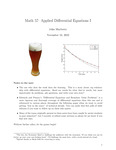## Pacific Open Texts

#### Title

Math 57: Applied Differential Equations I

#### Files

Full Text (2.8 MB)

#### Description

This book is designed for the fourth semester, “capstone” course in a calculus sequence with an emphasis on modeling with linear differential equations. Students will learn to translate verbal descriptions of physical problems into differential equation models, solve and visualize solutions to differential equations using MATLAB, calculate and investigate the behavior of analytic solutions to linear differential equations, discuss how solutions to differential equations depend on parameters, and interpret solutions to differential equations in the context of applications.

11-2022

#### Keywords

MATLAB, calculus, OER, Open Educational Resource, Modeling, Linear differential equations